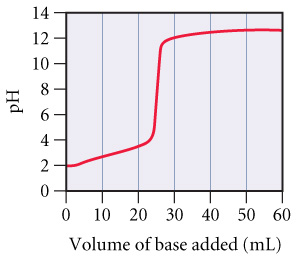# Problem: A 0.229-g sample of an unknown monoprotic acid was titrated with 0.112 M NaOH and the resulting titration curve is shown  in the figure.Determine the molar mass of the acid.

###### FREE Expert Solution

We’re being asked to calculate the molar mass of an unknown acid based on our interpretation of the titration curve

Recall that at the equivalence point of a titration:

88% (410 ratings)###### Problem Details

A 0.229-g sample of an unknown monoprotic acid was titrated with 0.112 M NaOH and the resulting titration curve is shown  in the figure.

Determine the molar mass of the acid.